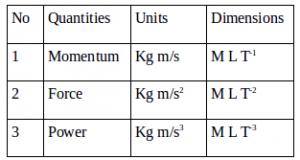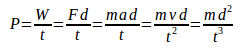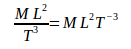# Physical quantities Units Dimensions – Problems and Solutions

3 Physical quantities Units Dimensions – Problems and Solutions

1.Based on the above table, which quantities have true units and dimensions.

Solution :

1) Momentum

The equation of momentum is p = m v

p = momentum, m = mass, v = velocity

Dimension of mass = M and dimension of velocity = L/T = L T-1 so that dimension of momentum = M L T-1

International unit of momentum = kg m/s = kg m s-1

2) Force

The equation of force is F = m a

F = force, m = mass, a = acceleration

Dimension of mass = M and dimension of acceleration = L/T2 = L T-2 so dimension of force is M L T-2

International unit of force is kg m/s2 = kg m s-2

3) Power

The equation of power is W = F d

W = work, F = force, d = displacement

Dimension of force = M L T-2 and dimension of displacement is L so that dimension of work is [M][L][T]-2 [L] = [M][L]2[T]-2

The equation of power is P = W / t

P = power, W = work, t = time

Dimension of work = [M][L]2[T]-2 and dimension of time = [T] so that dimension of power = [M][L]2[T]-2 / [T] = [M][L]2[T]-2 [T]-1 = [M][L]2[T]-3

International unit of force is kg m2/s3 = kg m2 s-3

[irp]

2. Based on table below, quantities with correct units and dimension are….Solution :

The equation of momentum is p = m v.

Unit of mass (m) is kilogram (kg) and unit of velocity (v) is meter per second (m/s) so that unit of momentum is kg m/s or kg m/s. Kilogram is the dimension of mass with dimension of [M], meter is a unit of length with a dimension of [L], second is the unit of time with dimension of [T] so that dimension of momentum is [M][L]/[T] or [M][L][T]-1.

The equation of force is F = m a.

Unit of Mass (m) is kilogram (kg) and unit of acceleration (a) is meters per second squared (m/s2) so the unit of force is kg m/s2 or kg m s-2. Unit of mass is kilogram with dimension of [M], unit of length is meter with dimension of [L], unit of time is second with dimension of [T] so that dimension of force is [M][L]/[T]2 or [M][L][T]-2

The equation of power is P = W/t, the equation of work is W = F s, the equation of force is F = m a.

Unit of mass is kilogram (kg), unit of acceleration is meters per second squared (m/s2) so that unit of force is kg m/s2. Unit of displacement is meter (m), unit of force is kg m/s2 so that unit of work is kg m/s2 x m = kg m2/s2. Unit of time is second (s), a unit of work is kg m2/s2 so that unit of power is kg m2/s2 : s = kg m2/s3 or kg m2 s-3.

Unit of mass is kilogram with dimension of [M], unit of length is meter with dimension of [L], unit of time is second with the dimension of [T] so that dimension of power is [M][L]2/[T]3 or [M][L]2[T]-3.

[irp]

3. Power is defined as the rate at which work is done. Or power is the ratio of work to the time interval. Determine the dimension of power.

Solution :

The equation of power :W = work, F = power, a = acceleration, v = velocity, d = distance, t = time interval

m = mass (dimension of mass = M), d = distance (dimension of distance = L), t = time (dimension of time = T).

Dimension of power :